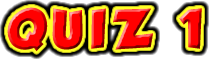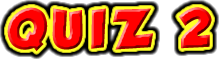ChaptersThis text is meant to accompany class discussions. It is not everything there is to know about Coulomb's Law. It is meant as a  prep for class. More detailed notes and examples are given in the class notes, presentations, and demonstrations (click here.)Click for the questions that go with this reading

• Math Background -Prefixes
• Figuring Forces Out Direction
• Free Body Diagram as an Equation
• Coulomb's Law
• Example: Charges in a Line

Introduction

Any number of particles with a net charge will feel either an attraction or repulsion from the other charges. This force is an electric force. This force is modeled by Coulomb's Law.

Math Background

In order to conserve space, scientist and engineers use a math short hand to represent certain powers of ten. The table below show the short hand and how it is used. (Learn these prefixes.)The display on a calculator can only display a specific number of digits across the screen before it runs out of room. Calculator manufactures wanted to create symbol the would use up less screen space when displaying exponents raised to a power of ten. They created a shorthand so calculators could "text" you the answer while being able to fit more meaningful numbers on the screen. The shorthand looks like this

6E-3

The "E" take the place of "x10" Do not write down "E-3" or "E" anything when showing your work. The "E" is text messaging shorthand. It is not an excepted math practice. Instead you should write the number as

6x10-3.

The basics

Figuring out the force between two charged particle is a two step process.

1. Figure out if the particles are attracted or repelled.
2. Calculate the magnitude of the force between the particles using Coulomb's Law.

Figuring Out DirectionTwo charges are separated by the distance shown. What is the force felt on the negative charge?

To figure out the direction is the electric force on a charge, use the main rule for the charge model,

"Opposites attract, likes repel."

Since these two charges have opposite signs, their forces will attract each other.The negative charge will feel a force pulling it to the left. The positive charge will feel and equal and opposite force pulling it to the right. Since the original question was about the negative charge, ignore what happens to positive, blue, charge. The magnitude of this force is calculated from Coulomb's Law.Free Body Diagrams as an Equation

To be successful at solving problems with multiple charges, free body diagrams must be mastered. The free body diagram will create the equation that is used to calculate the answer.Coulomb's Law & Calculating the Force's Magnitude Charles A. de Coulomb came up with a math model for describing the magnitude of the force of attraction between any two charges. The equation he came up with is referred to as Coulomb's Law and is shown below.Note that the q's are the charges' magnitudes. Do not put the negative signs in the equation. At this point plug in the numbers.A couple of things to note about the solution. The negative signs on the charges were dropped. This is because the equation is being used to calculate the magnitude of the force and not the direction. The direction was calculated in the first sectionabove by using the charge model's rule. Also note the givens are listed when solving the problem.
Implications of the Equation

Coulomb's Law's equation states that the force is directly proportional to the charges.This means if:

• One of the charges triples, then the force also triples. 3F = 3q1q2
• Both of the charges triple then the force changes by a factor of 9. 9F = 3q1 3q2
• If one charge doubles and the other triples, then the force changes by a factor of 6. 2F = 2q1 3q2

Coulomb's Law's equation also means that the force is inversely proportional to square the distance between the charges.This means if:

• The distance between two charges is doubled then the force changes by a factor of 1/4. (1/22)=1/4
• If the charges get closer to each other, distance changes by a factor of 1/3 then the force changes by a factor of 9. (1/32)=9.
• If the force between two charges a factor 16, then the distance between the charges changed by a factor of 1/4. (1/r2)=16, r=4
• If the force between two charges a factor 1/4, then the distance between between the charges changed by a factor of 2. (1/r2)=(1/4), r=2
• If the distance between the charges changes by a factor of 5/3, then the force changes by a factor of 9/25.Example What happens to the force if one charge is tripled, other charge is halved, and the distance between them is tripled?

Example Problem: Multiple Charges in a Line
• Question
• Hint
• Solution
• Video SolutionFind the net force on charge "B."

 Quick overview of the solution steps. Identify the directions of the forces acting on the particle in question using the charge model's rule that opposites attract and likes repel. Create a free body diagram from these forces. IF the charges do not ALL line up in a straight line, find the vertical and horizontal components of each force and combine the components by summing up the forces in the "X" and then the "Y" directions. Find the magnitude of each force. This means to ignore the plus and minus signs on the charges when doing the math. Plug in the magnitudes into the equation created from the free body. Solve.3

by Tony Wayne ...(If you are a teacher, please feel free to use these resources in your teaching.)